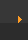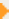## Stoichiometry: Moles to Grams (1 of 5)

Converting from moles to grams is one of those things in chemistry that has to be automatic. The good thing is once you understand the pattern, mole to gram conversions are all done the same way (just change the GFM to that of the molecule you are using in the problem).

Moles to Grams Step-by-Step Example: Convert 3.5 moles of H2O to grams. (watch video)

1. Circle and label what you are given and what you are trying to find.
Given: 3.5 moles of H2O. Trying to find: number of grams of H2O.
2. Find the GFM for the substance (in this case H2O). For water the GFM is 18.02 g/mol.
3. Set up the math in the following format:
3.5 moles H2O    x    18.02 grams/1 mole H2O
4. Cancel out units and multiply.
3.5 moles H2O    x    18.02 grams/1 mole H2O    =    3.5 x 18.02 grams    =    63.07 grams
5. Check to see if you answer makes sense. Here we know that 1 mole of H2O is about 20 grams (look at the GFM). So 3.5 moles should be about three times more—a bit over 60 grams. Therefore our answer makes sense.

Remember: when converting moles to grams: multiply by the GFM.

Try one. Convert 10.31 moles of H2O to grams. (watch video)## Moles to Grams: Some Notes

When you are converting between moles and grams you aren't changing the quantities, just the units. If I put one mole of H2O on a balance it will have a mass of 18.02g. If I put half a mole on the balance it will weigh half as much (9.01 grams). The periodic table tells me how much one mole of each element weighs. Once I know this I can convert moles to grams and then check to see if my answer makes sense. If I have one mole of carbon (the periodic table shows one mole of carbon to have a mass of 12.01 grams) and I want to know the mass of 0.33 moles my answer had better be less than 12.01 grams or it won't make sense.

 Next pageThe GFM for water can be written as:

18.02 grams/mole H2O or

18.02 g/mol H2O or

18.02 grams/1 mole H2O

They all mean the same thing.

Help calculating the GFM